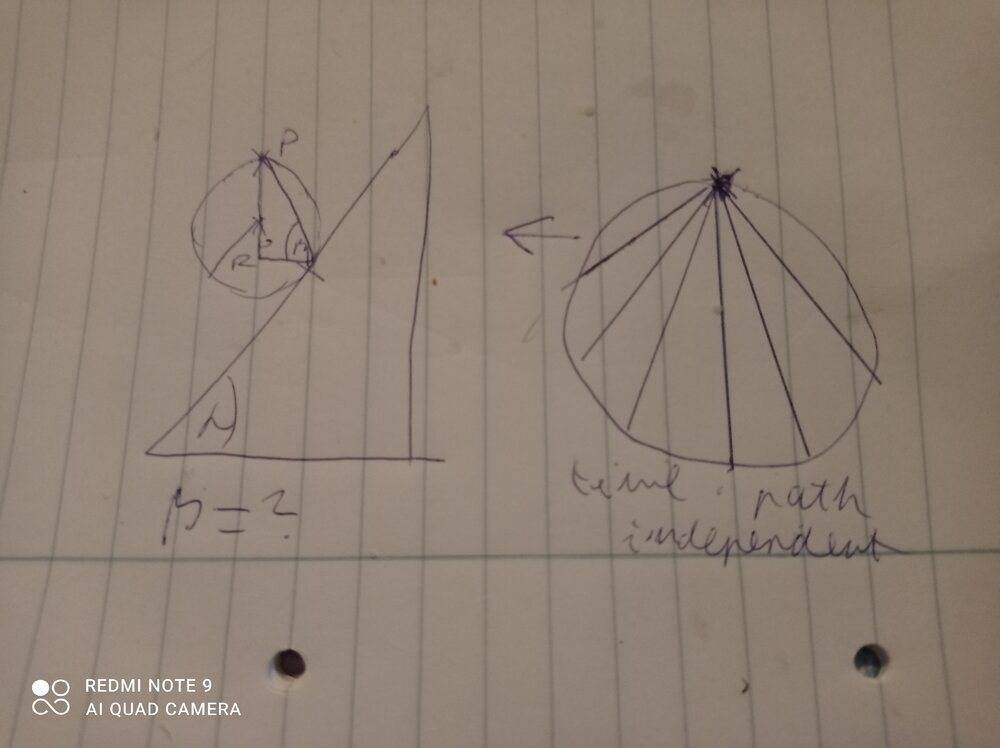# Path that requires the least time to travel along

bbal
Homework Statement:
We have slope, over which there's a point (P). The point is connected to the slope with a straight line. Find the line, a small ball would travel along the fastest.
Relevant Equations:
S=(at^2)/2Gold Member

•Frigus
bbal
•